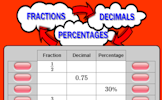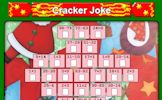# Fraction, decimal and percentage equivalence

### Term 1 starting in week 10 :: Estimated time: 3 weeks

• Represent tenths and hundredths as diagrams
• Represent tenths and hundredths on number lines
• Interchange between fractional and decimal number lines
• Convert between fractions and decimals - tenths and hundredths
• Convert between fractions and decimals - fifths and quarters
• Convert between fractions and decimals — eighths and thousandths
• Understand the meaning of percentage using a hundred square
• Convert fluently between simple fractions, decimals and percentages
• Use and interpret pie charts
• Represent any fraction as a diagram
• Represent fractions on number lines
• Identify and use simple equivalent fractions
• Understand fractions as division
• Convert fluently being fractions, decimals and percentages
• Explore fractions above one, decimals and percentages

This page should remember your ticks from one visit to the next for a period of time. It does this by using Local Storage so the information is saved only on the computer you are working on right now.

## Lesson Starters

Here are some suggestions for whole-class, projectable resources which can be used at the beginnings of each lesson in this block.

### 1st Lesson#### Fractions Decimals Percentages

Convert fractions to decimals, decimals to percentages and percentages to fractions.

### 2nd Lesson#### PercenTable

Complete the table by calculating common percentages without using a calculator.

### 3rd Lesson#### Estimating Percentages

Estimate the percentages of full circles and rectangles the sectors represent.

### 4th Lesson#### Christmas Eve Snow

Each different letter stands for a different digit. Can you make sense of this word sum?

### 5th Lesson#### Puddings

Complete the calculations with the weights of the puddings

### 6th Lesson#### Christmas Bells

If all the bells ring together at noon, at what time will they next all ring together? This problem requires the use of LCM.

### 7th Lesson#### Christmas Presents

Work out the total cost of five Christmas presents from the information given.

### 8th Lesson#### Cracker Joke

Answer the mental arithmetic questions then convert the answers to letters to find the joke.

### 9th Lesson#### Twelve Days

A Maths puzzle based on the 12 Days of Christmas song.

Some of the Starters above are to reinforce concepts learnt, others are to introduce new ideas while others are on unrelated topics designed for retrieval practice or and opportunity to develop problem-solving skills.

White Rose ResourcesEnd of block assessments provide a quick progress check at the end of each block of learning to make sure students have understood the content covered. This Scheme of Learning was produced by White Rose Maths and is used here with permission granted on 30th June 2021.For All: